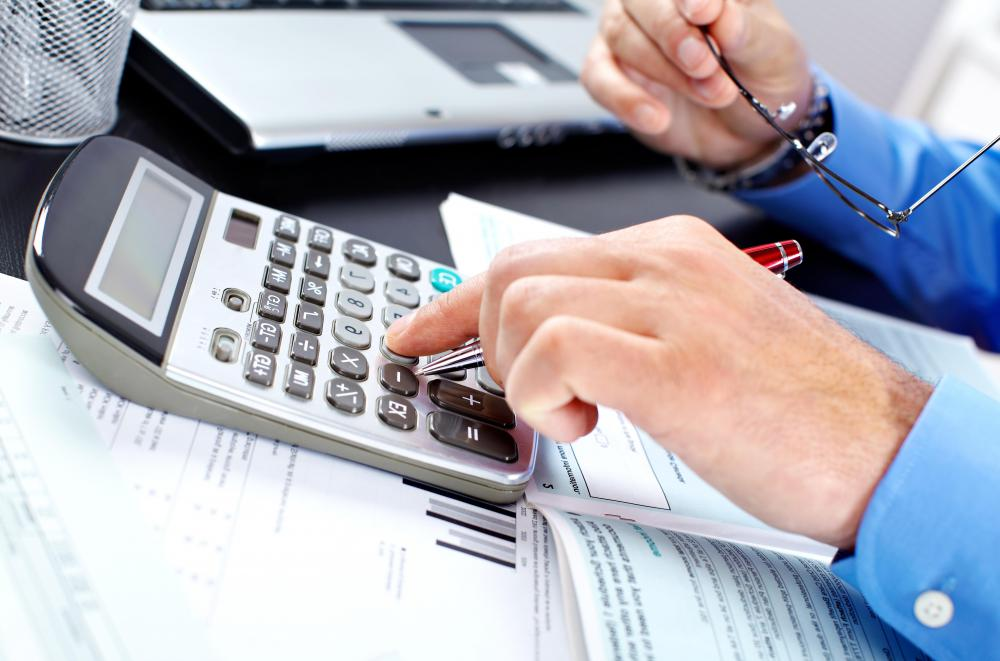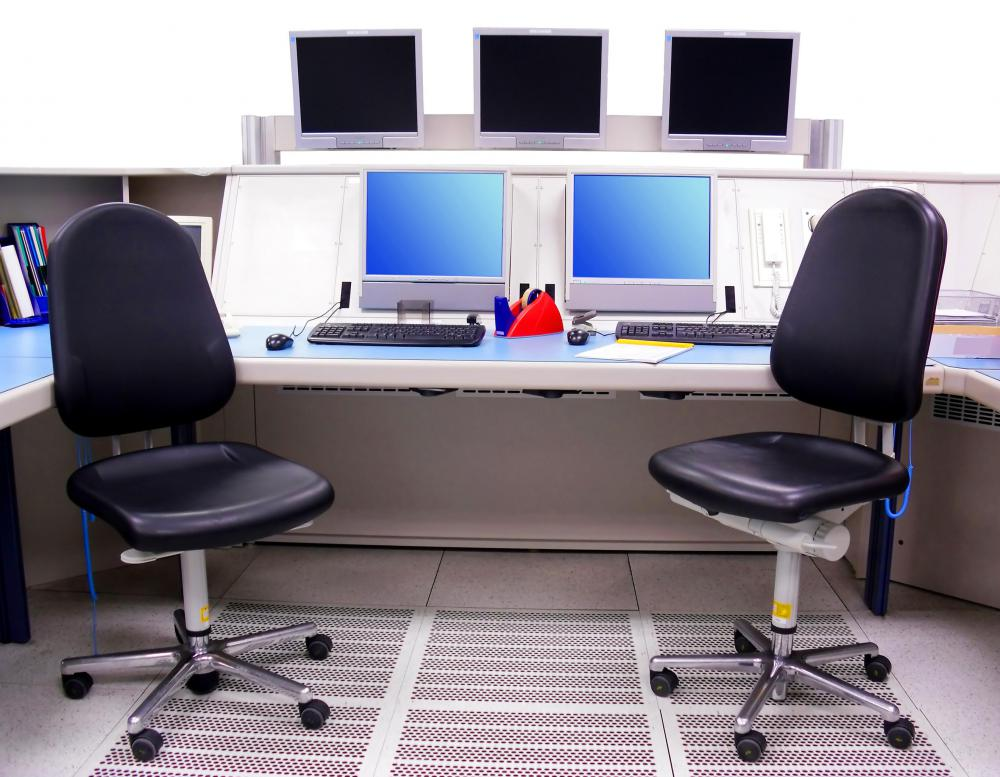# How do I Calculate Computer Depreciation? (with pictures)

Stacy C.

Basic computer depreciation, which is how much monetary value a computer has lost over time, can be figured two ways: the straight-line method and the declining-balance method. The straight-line method assumes the value of the computer decreases by the same amount every year, while the declining-balance method has the computer losing more value when it is newer and less value as it ages. Either of these methods can be used to calculate computer value, which is typically done for tax purposes.The straight-line calculation method to calculate computer depreciation assumes value decreases by the same out each year.

In the the straight-line method of calculating computer depreciation, just two pieces of information are needed: the price of the computer when it was originally purchased and how many years it has been since that time. For the first year, take the original purchase price and multiply it by 20%. Subtract that number from the purchase price, and the resulting number is how much the computer is worth after one year of use. For example, if a computer cost \$1,000 US Dollars (USD), multiplying it by 20% is \$200. \$1,000 - \$200 = \$800. The value of the computer after one year of use is then \$800.To claim depreciation on tax returns, the computer equipment must be owned by the company and used for business activity that generates income.

To calculate subsequent years, the first year’s percentage must be used. Using the \$1,000 example, year two would be calculated by taking the computer’s value after the first year, \$800, and subtracting another \$200, making the computer worth \$600 after the second year. The third year would result in a value of \$400, the fourth year would be \$200, and by the fifth year the computer has depreciated entirely, to a value of zero.

The declining-balance method to figure computer depreciation is a bit more complicated. The first step is the same: start with the computer’s original value, but then multiply that by 40%. For a \$1,000 computer, this is \$400. Subtract the amount from the original price of the computer, giving the computer a value of \$600 after the first year. For the second year, take the previous year's value, in this case \$600, times 40%, which is \$240. Subtract \$240 from \$600, giving a value of \$360 after the second year.

In the U.S., in order to claim computer depreciation on taxes, the claimer must own the computer, use it for a business-related venture or activity that generates income, and it must have a useful life of more than one year. Form 4562 must be used when declaring depreciated items on a federal tax return. More information on this procedure can be obtained from the Internal Revenue Service (IRS).

## You might also Likeanon956462

It's flawed, though. Your computer won't be worth anything after four years. I dug up an old computer and had people offering to pay more than I asked. Consider parting out older ones. You can get more money for them, but it is more work. You may be surprised how many people like to tinker with older parts.

Markerrag

I'd argue the declining-balance method is the most accurate because computers lose their value in a hurry as technology advances. Just ask anyone who has bought a top of the line machine what happens to it in a few months -- that's right, it's merely a good computer that has been bested by something newer and faster.

When it comes to the value of assets for tax purposes, there are some obvious advantages with the declining-balance method because companies tend to buy a lot of computers at once. The tax hit will be less with the declining-balance method and one can justify using it, too.